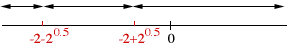the question is: on what interval is f(x)=(x2)(ex)? ive found the 2nd derivative which is ex(x2+4x+2) and i did the quadratic to get -2-20.5 and -2+20.5, but i dont know what the interval is. can u please help quickly? Hi Alex, You didn't say what interval you are looking for but, since you have taken the second derivative, I expect that you are looking for the interval(s) where f(x) is concave upwards or concave downwards. You have done most of the work. You found that the second derivative is zero at -2-20.5 and -2+20.5. These are the only points where the second derivative could change from positive to negative or negative to positive. The two points divide the line into three intervals.the interval from minus infinity to -2-20.5 the interval from -2-20.5 to -2+20.5 the interval from -2+20.5 to infinity All that remains is to check each of the three intervals to see in which the curve is concave upwards and in which the curve is concave downwards. I'll do one, the interval from minus infinity to -2-20.5. Choose some point in the interval. Since -2-20.5 is approximately -3.41, I am going to choose -5. Find the sign of f''(-5). f''(-5) = e-5((-5)2 + 4(-5) + 2) > 0. Since f''(-5) > 0, f(x) is concave upwards at -5 and thus concave upwards in the interval from minus infinity to -2-20.5 I hope this helps, Harley Go to Math Central